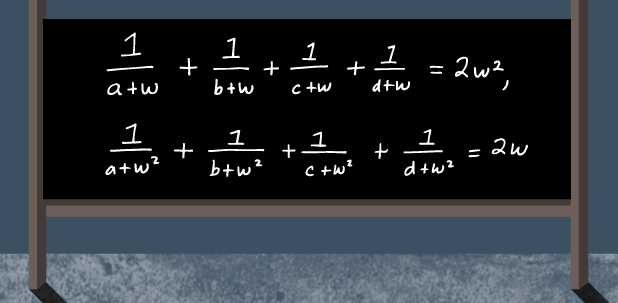# Too Complex! Part- II

Algebra Level 3If Real numbers a, b, c and d (none of which are $-1$ ) satisfy the following conditions:

$\frac{1}{a+ ω} + \frac{1}{b+ ω}+ \frac{1}{c+ ω} + \frac{1}{d+ ω} = 2 ω^{2},$ $\frac{1}{a+ ω^{2}}+ \frac{1}{b+ ω^{2}} + \frac{1}{c+ ω^{2}} + \frac{1}{d+ ω^{2}} = 2 ω.$

To 2 decimal places, find the value of -

$\frac{1}{a+ 1} + \frac{1}{b+ 1}+ \frac{1}{c+ 1} + \frac{1}{d+ 1}$

Note that ω and $ω^{2}$ are the complex cube roots of unity other than 1.

×